# electromagnetic induction ( part 1 )

2. Aug 2021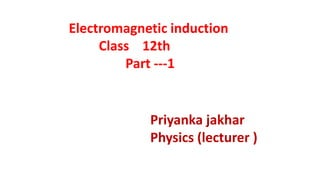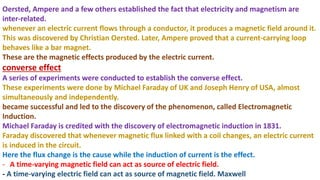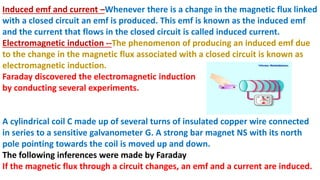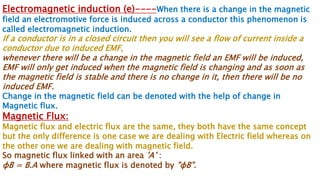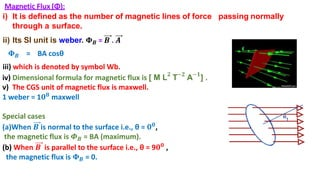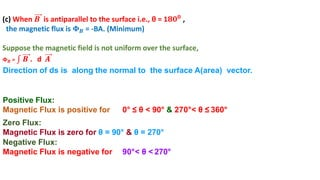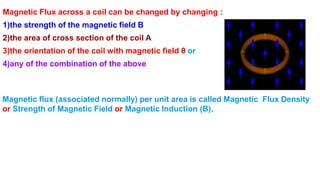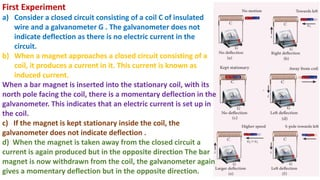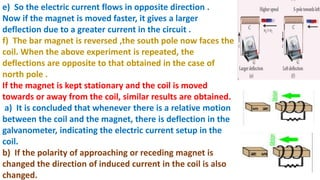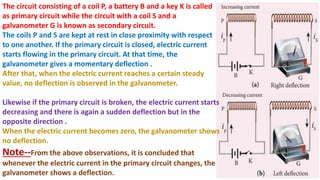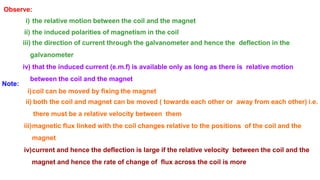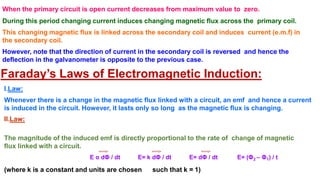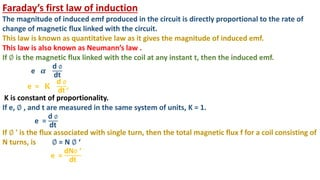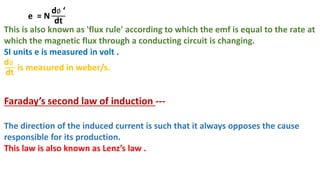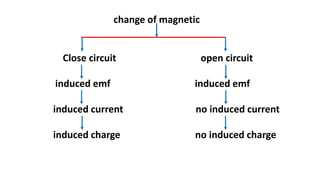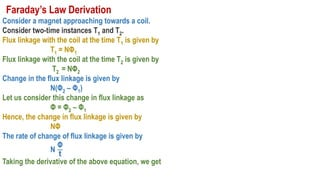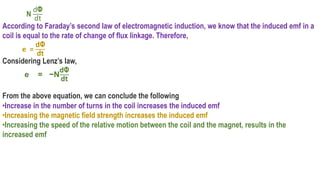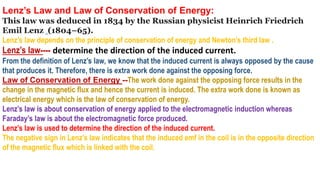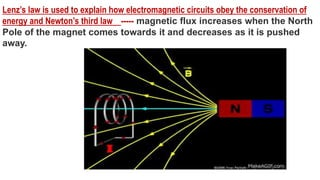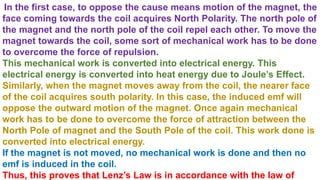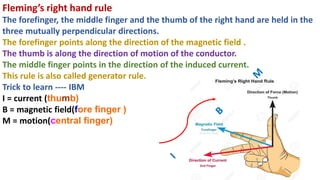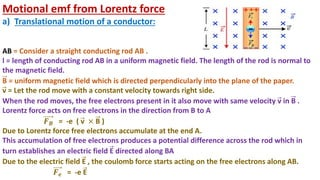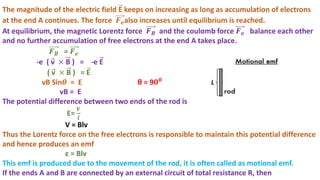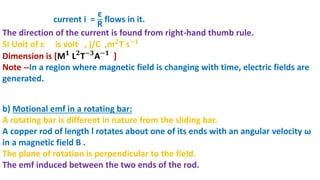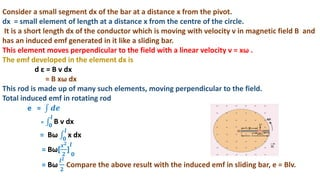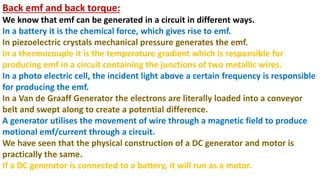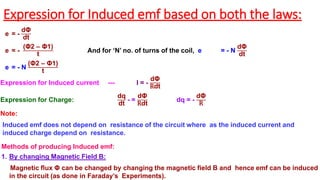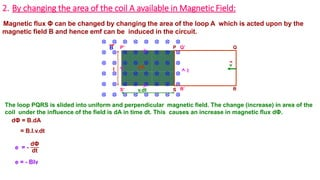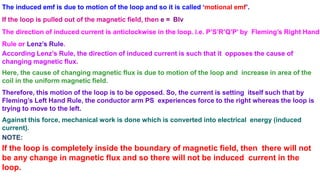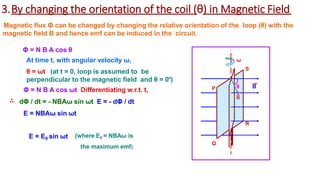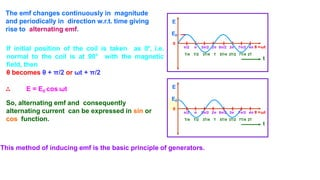1 von 31

### electromagnetic induction ( part 1 )

• 1. Electromagnetic induction Class 12th Part ---1 Priyanka jakhar Physics (lecturer )
• 2. Oersted, Ampere and a few others established the fact that electricity and magnetism are inter-related. whenever an electric current flows through a conductor, it produces a magnetic field around it. This was discovered by Christian Oersted. Later, Ampere proved that a current-carrying loop behaves like a bar magnet. These are the magnetic effects produced by the electric current. converse effect A series of experiments were conducted to establish the converse effect. These experiments were done by Michael Faraday of UK and Joseph Henry of USA, almost simultaneously and independently. became successful and led to the discovery of the phenomenon, called Electromagnetic Induction. Michael Faraday is credited with the discovery of electromagnetic induction in 1831. Faraday discovered that whenever magnetic flux linked with a coil changes, an electric current is induced in the circuit. Here the flux change is the cause while the induction of current is the effect. - A time-varying magnetic field can act as source of electric field. - A time-varying electric field can act as source of magnetic field. Maxwell
• 3. Induced emf and current –Whenever there is a change in the magnetic flux linked with a closed circuit an emf is produced. This emf is known as the induced emf and the current that flows in the closed circuit is called induced current. Electromagnetic induction --The phenomenon of producing an induced emf due to the change in the magnetic flux associated with a closed circuit is known as electromagnetic induction. Faraday discovered the electromagnetic induction by conducting several experiments. A cylindrical coil C made up of several turns of insulated copper wire connected in series to a sensitive galvanometer G. A strong bar magnet NS with its north pole pointing towards the coil is moved up and down. The following inferences were made by Faraday If the magnetic flux through a circuit changes, an emf and a current are induced.
• 4. Electromagnetic induction (e)----When there is a change in the magnetic field an electromotive force is induced across a conductor this phenomenon is called electromagnetic induction. If a conductor is in a closed circuit then you will see a flow of current inside a conductor due to induced EMF, whenever there will be a change in the magnetic field an EMF will be induced, EMF will only get induced when the magnetic field is changing and as soon as the magnetic field is stable and there is no change in it, then there will be no induced EMF. Change in the magnetic field can be denoted with the help of change in Magnetic flux. Magnetic Flux: Magnetic flux and electric flux are the same, they both have the same concept but the only difference is one case we are dealing with Electric field whereas on the other one we are dealing with magnetic field. So magnetic flux linked with an area 'A’ : ɸB = B.A where magnetic flux is denoted by "ɸB".
• 5. Magnetic Flux (Φ): i) It is defined as the number of magnetic lines of force passing normally through a surface. ii) Its SI unit is weber. 𝚽𝑩 = 𝑩 . 𝑨 𝚽𝑩 = BA cosθ iii) which is denoted by symbol Wb. iv) Dimensional formula for magnetic flux is [ M L𝟐 T−𝟐 A−𝟏 ] . v) The CGS unit of magnetic flux is maxwell. 1 weber = 1𝟎𝟖 maxwell Special cases (a)When 𝑩 is normal to the surface i.e., θ = 𝟎𝟎, the magnetic flux is 𝜱𝑩 = BA (maximum). (b) When 𝑩 is parallel to the surface i.e., θ = 9𝟎𝟎 , the magnetic flux is 𝚽𝑩 = 0.
• 6. (c) When 𝑩 is antiparallel to the surface i.e., θ = 1𝟖𝟎𝟎 , the magnetic flux is 𝚽𝑩 = -BA. (Minimum) Suppose the magnetic field is not uniform over the surface, 𝚽𝑩 = 𝑩 . d 𝑨 Direction of ds is along the normal to the surface A(area) vector. Positive Flux: Magnetic Flux is positive for 0° ≤ θ < 90° & 270°< θ ≤ 360° Zero Flux: Magnetic Flux is zero for θ = 90° & θ = 270° Negative Flux: Magnetic Flux is negative for 90°< θ < 270°
• 7. Magnetic Flux across a coil can be changed by changing : 1)the strength of the magnetic field B 2)the area of cross section of the coil A 3)the orientation of the coil with magnetic field θ or 4)any of the combination of the above Magnetic flux (associated normally) per unit area is called Magnetic Flux Density or Strength of Magnetic Field or Magnetic Induction (B).
• 8. First Experiment a) Consider a closed circuit consisting of a coil C of insulated wire and a galvanometer G . The galvanometer does not indicate deflection as there is no electric current in the circuit. b) When a magnet approaches a closed circuit consisting of a coil, it produces a current in it. This current is known as induced current. When a bar magnet is inserted into the stationary coil, with its north pole facing the coil, there is a momentary deflection in the galvanometer. This indicates that an electric current is set up in the coil. c) If the magnet is kept stationary inside the coil, the galvanometer does not indicate deflection . d) When the magnet is taken away from the closed circuit a current is again produced but in the opposite direction The bar magnet is now withdrawn from the coil, the galvanometer again gives a momentary deflection but in the opposite direction.
• 9. e) So the electric current flows in opposite direction . Now if the magnet is moved faster, it gives a larger deflection due to a greater current in the circuit . f) The bar magnet is reversed ,the south pole now faces the coil. When the above experiment is repeated, the deflections are opposite to that obtained in the case of north pole . If the magnet is kept stationary and the coil is moved towards or away from the coil, similar results are obtained. a) It is concluded that whenever there is a relative motion between the coil and the magnet, there is deflection in the galvanometer, indicating the electric current setup in the coil. b) If the polarity of approaching or receding magnet is changed the direction of induced current in the coil is also changed.
• 10. The circuit consisting of a coil P, a battery B and a key K is called as primary circuit while the circuit with a coil S and a galvanometer G is known as secondary circuit. The coils P and S are kept at rest in close proximity with respect to one another. If the primary circuit is closed, electric current starts flowing in the primary circuit. At that time, the galvanometer gives a momentary deflection . After that, when the electric current reaches a certain steady value, no deflection is observed in the galvanometer. Likewise if the primary circuit is broken, the electric current starts decreasing and there is again a sudden deflection but in the opposite direction . When the electric current becomes zero, the galvanometer shows no deflection. Note--From the above observations, it is concluded that whenever the electric current in the primary circuit changes, the galvanometer shows a deflection.
• 11. Observe: i) the relative motion between the coil and the magnet ii) the induced polarities of magnetism in the coil iii) the direction of current through the galvanometer and hence the deflection in the galvanometer iv) that the induced current (e.m.f) is available only as long as there is relative motion between the coil and the magnet Note: i)coil can be moved by fixing the magnet ii) both the coil and magnet can be moved ( towards each other or away from each other) i.e. there must be a relative velocity between them iii)magnetic flux linked with the coil changes relative to the positions of the coil and the magnet iv)current and hence the deflection is large if the relative velocity between the coil and the magnet and hence the rate of change of flux across the coil is more
• 12. When the primary circuit is open current decreases from maximum value to zero. During this period changing current induces changing magnetic flux across the primary coil. This changing magnetic flux is linked across the secondary coil and induces current (e.m.f) in the secondary coil. However, note that the direction of current in the secondary coil is reversed and hence the deflection in the galvanometer is opposite to the previous case. Faraday’s Laws of Electromagnetic Induction: I.Law: Whenever there is a change in the magnetic flux linked with a circuit, an emf and hence a current is induced in the circuit. However, it lasts only so long as the magnetic flux is changing. II.Law: The magnitude of the induced emf is directly proportional to the rate of change of magnetic flux linked with a circuit. (where k is a constant and units are chosen such that k = 1) E α dΦ / dt E= k dΦ / dt E= dΦ / dt E= (Φ2 – Φ1) / t
• 13. Faraday’s first law of induction The magnitude of induced emf produced in the circuit is directly proportional to the rate of change of magnetic flux linked with the circuit. This law is known as quantitative law as it gives the magnitude of induced emf. This law is also known as Neumann’s law . If ∅ is the magnetic flux linked with the coil at any instant t, then the induced emf. e 𝜶 d ∅ dt e = 𝐊 d ∅ dt , K is constant of proportionality. If e, ∅ , and t are measured in the same system of units, K = 1. e = d ∅ dt If ∅ ' is the flux associated with single turn, then the total magnetic flux f for a coil consisting of N turns, is ∅ = N ∅ ‘ e = dN∅ ‘ dt
• 14. e = N d∅ ‘ dt This is also known as 'flux rule' according to which the emf is equal to the rate at which the magnetic flux through a conducting circuit is changing. SI units e is measured in volt . d∅ dt is measured in weber/s. Faraday’s second law of induction --- The direction of the induced current is such that it always opposes the cause responsible for its production. This law is also known as Lenz’s law .
• 15. change of magnetic Close circuit open circuit induced emf induced emf induced current no induced current induced charge no induced charge
• 16. Faraday’s Law Derivation Consider a magnet approaching towards a coil. Consider two-time instances T1 and T2. Flux linkage with the coil at the time T1 is given by T1 = NΦ1 Flux linkage with the coil at the time T2 is given by T2 = NΦ2 Change in the flux linkage is given by N(Φ2 – Φ1) Let us consider this change in flux linkage as Φ = Φ2 – Φ1 Hence, the change in flux linkage is given by NΦ The rate of change of flux linkage is given by N Φ t Taking the derivative of the above equation, we get
• 17. N dΦ dt According to Faraday’s second law of electromagnetic induction, we know that the induced emf in a coil is equal to the rate of change of flux linkage. Therefore, e = dΦ dt Considering Lenz’s law, e = −N dΦ dt From the above equation, we can conclude the following •Increase in the number of turns in the coil increases the induced emf •Increasing the magnetic field strength increases the induced emf •Increasing the speed of the relative motion between the coil and the magnet, results in the increased emf
• 18. Lenz’s Law and Law of Conservation of Energy: This law was deduced in 1834 by the Russian physicist Heinrich Friedrich Emil Lenz (1804–65). Lenz’s law depends on the principle of conservation of energy and Newton’s third law . Lenz’s law---- determine the direction of the induced current. From the definition of Lenz’s law, we know that the induced current is always opposed by the cause that produces it. Therefore, there is extra work done against the opposing force. Law of Conservation of Energy --The work done against the opposing force results in the change in the magnetic flux and hence the current is induced. The extra work done is known as electrical energy which is the law of conservation of energy. Lenz’s law is about conservation of energy applied to the electromagnetic induction whereas Faraday’s law is about the electromagnetic force produced. Lenz’s law is used to determine the direction of the induced current. The negative sign in Lenz’s law indicates that the induced emf in the coil is in the opposite direction of the magnetic flux which is linked with the coil.
• 19. Lenz’s law is used to explain how electromagnetic circuits obey the conservation of energy and Newton’s third law ----- magnetic flux increases when the North Pole of the magnet comes towards it and decreases as it is pushed away.
• 20. In the first case, to oppose the cause means motion of the magnet, the face coming towards the coil acquires North Polarity. The north pole of the magnet and the north pole of the coil repel each other. To move the magnet towards the coil, some sort of mechanical work has to be done to overcome the force of repulsion. This mechanical work is converted into electrical energy. This electrical energy is converted into heat energy due to Joule’s Effect. Similarly, when the magnet moves away from the coil, the nearer face of the coil acquires south polarity. In this case, the induced emf will oppose the outward motion of the magnet. Once again mechanical work has to be done to overcome the force of attraction between the North Pole of magnet and the South Pole of the coil. This work done is converted into electrical energy. If the magnet is not moved, no mechanical work is done and then no emf is induced in the coil. Thus, this proves that Lenz’s Law is in accordance with the law of
• 21. Fleming’s right hand rule The forefinger, the middle finger and the thumb of the right hand are held in the three mutually perpendicular directions. The forefinger points along the direction of the magnetic field . The thumb is along the direction of motion of the conductor. The middle finger points in the direction of the induced current. This rule is also called generator rule. Trick to learn ---- IBM I = current (thumb) B = magnetic field(fore finger ) M = motion(central finger)
• 22. Motional emf from Lorentz force a) Translational motion of a conductor: AB = Consider a straight conducting rod AB . l = length of conducting rod AB in a uniform magnetic field. The length of the rod is normal to the magnetic field. B = uniform magnetic field which is directed perpendicularly into the plane of the paper. v = Let the rod move with a constant velocity towards right side. When the rod moves, the free electrons present in it also move with same velocity v in B . Lorentz force acts on free electrons in the direction from B to A 𝑭𝑩 = -e ( v × B ) Due to Lorentz force free electrons accumulate at the end A. This accumulation of free electrons produces a potential difference across the rod which in turn establishes an electric field E directed along BA Due to the electric field E , the coulomb force starts acting on the free electrons along AB. 𝑭𝒆 = -e E
• 23. The magnitude of the electric field E keeps on increasing as long as accumulation of electrons at the end A continues. The force 𝑭𝒆also increases until equilibrium is reached. At equilibrium, the magnetic Lorentz force 𝑭𝑩 and the coulomb force 𝑭𝒆 balance each other and no further accumulation of free electrons at the end A takes place. 𝑭𝑩 = 𝑭𝒆 -e ( v × B ) = -e E ( v × B ) = E vB Sin𝜽 = E θ = 9𝟎𝟎 vB = E The potential difference between two ends of the rod is E= 𝑽 𝒍 V = Blv Thus the Lorentz force on the free electrons is responsible to maintain this potential difference and hence produces an emf ε = Blv This emf is produced due to the movement of the rod, it is often called as motional emf. If the ends A and B are connected by an external circuit of total resistance R, then
• 24. current i = ε R flows in it. The direction of the current is found from right-hand thumb rule. SI Unit of ε is volt , j/C ,m𝟐T s−𝟏 Dimension is [M𝟏 L𝟐 T−𝟑 A−𝟏 ] Note --In a region where magnetic field is changing with time, electric fields are generated. b) Motional emf in a rotating bar: A rotating bar is different in nature from the sliding bar. A copper rod of length l rotates about one of its ends with an angular velocity ω in a magnetic field B . The plane of rotation is perpendicular to the field. The emf induced between the two ends of the rod.
• 25. Consider a small segment dx of the bar at a distance x from the pivot. dx = small element of length at a distance x from the centre of the circle. It is a short length dx of the conductor which is moving with velocity v in magnetic field B and has an induced emf generated in it like a sliding bar. This element moves perpendicular to the field with a linear velocity v = xω . The emf developed in the element dx is d ε = B v dx = B xω dx This rod is made up of many such elements, moving perpendicular to the field. Total induced emf in rotating rod e = 𝒅𝒆 = 𝟎 𝒍 B v dx = Bω 𝟎 𝒍 x dx = Bω[ 𝒙𝟐 𝟐 ] = Bω 𝒍𝟐 𝟐 Compare the above result with the induced emf in sliding bar, e = Blv. 𝒍 𝟎
• 26. Back emf and back torque: We know that emf can be generated in a circuit in different ways. In a battery it is the chemical force, which gives rise to emf. In piezoelectric crystals mechanical pressure generates the emf. In a thermocouple it is the temperature gradient which is responsible for producing emf in a circuit containing the junctions of two metallic wires. In a photo electric cell, the incident light above a certain frequency is responsible for producing the emf. In a Van de Graaff Generator the electrons are literally loaded into a conveyor belt and swept along to create a potential difference. A generator utilises the movement of wire through a magnetic field to produce motional emf/current through a circuit. We have seen that the physical construction of a DC generator and motor is practically the same. If a DC generator is connected to a battery, it will run as a motor.
• 27. Expression for Induced emf based on both the laws: e = - dΦ dt e = - (Φ2 – Φ1) t And for ‘N’ no. of turns of the coil, e = - N dΦ dt e = - N (Φ2 – Φ1) t Expression for Induced current --- I = - dΦ Rdt Expression for Charge: dq dt = - dΦ Rdt dq = - dΦ R Note: Induced emf does not depend on resistance of the circuit where as the induced current and induced charge depend on resistance. Methods of producing Induced emf: 1. By changing Magnetic Field B: Magnetic flux Φ can be changed by changing the magnetic field B and hence emf can be induced in the circuit (as done in Faraday’s Experiments).
• 28. 2. By changing the area of the coil A available in Magnetic Field: Magnetic flux Φ can be changed by changing the area of the loop A which is acted upon by the magnetic field B and hence emf can be induced in the circuit. S R P’ P Q’ Q S’ R’ v B dA l v.dt I The loop PQRS is slided into uniform and perpendicular magnetic field. The change (increase) in area of the coil under the influence of the field is dA in time dt. This causes an increase in magnetic flux dΦ. dΦ = B.dA = B.l.v.dt e = - dΦ dt e = - Blv
• 29. The induced emf is due to motion of the loop and so it is called ‘motional emf’. If the loop is pulled out of the magnetic field, then e = Blv The direction of induced current is anticlockwise in the loop. i.e. P’S’R’Q’P’ by Fleming’s Right Hand Rule or Lenz’s Rule. According Lenz’s Rule, the direction of induced current is such that it opposes the cause of changing magnetic flux. Here, the cause of changing magnetic flux is due to motion of the loop and increase in area of the coil in the uniform magnetic field. Therefore, this motion of the loop is to be opposed. So, the current is setting itself such that by Fleming’s Left Hand Rule, the conductor arm PS experiences force to the right whereas the loop is trying to move to the left. Against this force, mechanical work is done which is converted into electrical energy (induced current). NOTE: If the loop is completely inside the boundary of magnetic field, then there will not be any change in magnetic flux and so there will not be induced current in the loop.
• 30. 3.By changing the orientation of the coil (θ) in Magnetic Field: Magnetic flux Φ can be changed by changing the relative orientation of the loop (θ) with the magnetic field B and hence emf can be induced in the circuit. P Q R S B θ ω Φ = N B A cos θ At time t, with angular velocity ω, θ = ωt (at t = 0, loop is assumed to be perpendicular to the magnetic field and θ = 0°) Φ = N B A cos ωt Differentiating w.r.t. t, dΦ / dt = - NBAω sin ωt E = - dΦ / dt E = NBAω sin ωt E = E0 sin ωt (where E0 = NBAω is the maximum emf) n
• 31. The emf changes continuously in magnitude and periodically in direction w.r.t. time giving rise to alternating emf. If initial position of the coil is taken as 0°, i.e. normal to the coil is at 90° with the magnetic field, then θ becomes θ + π/2 or ωt + π/2 E = E0 cos ωt So, alternating emf and consequently alternating current can be expressed in sin or cos function. This method of inducing emf is the basic principle of generators. E T/2 3T/4 T 5T/4 3T/2 7T/4 2T t E0 0 π/2 π 3π/2 2π 5π/2 3π 7π/2 4π θ =ωt T/4 E T/2 3T/4 T 5T/4 3T/2 7T/4 2T t E0 0 π/2 π 3π/2 2π 5π/2 3π 7π/2 4π θ =ωt T/4